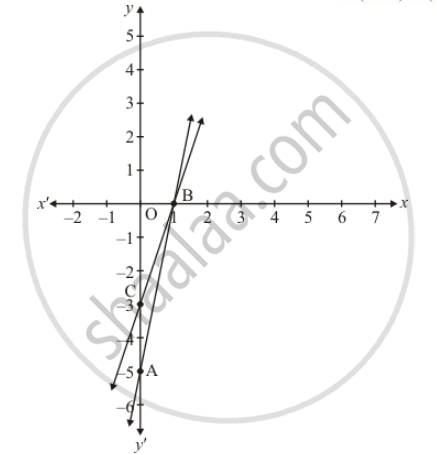# Draw the graphs of the equations 5x − y = 5 and 3x − y = 3. Determine the co-ordinates of the vertices of the triangle formed by these lines and the y axis. - Mathematics

Sum

Draw the graphs of the equations 5x − y = 5 and 3x − y = 3. Determine the coordinates of the vertices of the triangle formed by these lines and the y axis.

Draw the graphs of the equations 5x − y = 5 and 3x − y = 3. Determine the coordinates of the vertices of the triangle formed by these lines and y-axis. Calculate the area of the triangle so formed.

#### Solution

The given equations are:

5x − y = 5 ........(i)

3x − y = 3 ...........(ii)

Putting x = 0 in equation (i) we get

=> 5 xx 0 - y = 5

=> y = -5

x = 0, y = -5

Putting y = 0 in eqaution (i) we get

=> 5x - 0 = 5

=> x = 1

x = 1, y = 0

Use the following table to draw the graph.

 x 0 1 y -5 0

Draw the graph by plotting the two points A(0, -5), B(1, 0) from table3x - y = 3 ....(ii)

Putting x = 0 in eqaution (ii) we get

=> 3xx0-y = 3

=> y = -3

x= 0, y = -3

Putting y = 0 in equation (ii) we get

=> 3x - 0 = 3

=> x = 1`

x = 1, y = 0

Use the following table to draw the graph.

 x 0 1 y -3 0

Draw the graph by plotting the two points C(0, -3), D(1,0) from table.

Hence the vertices of the required triangle are B(1,0), C(0, -3) and A(0, -5).

Now,

Required area = Area of PCA

Required area = 1/2 (base x height)

Required area = 1/2 (2 x 1) sq.units

Hence the required area is 1 sq units

Concept: Equations Reducible to a Pair of Linear Equations in Two Variables
Is there an error in this question or solution?

#### APPEARS IN

NCERT Class 10 Maths
Chapter 3 Pair of Linear Equations in Two Variables
Exercise 3.7 | Q 6 | Page 68
RD Sharma Class 10 Maths
Chapter 3 Pair of Linear Equations in Two Variables
Exercise 3.2 | Q 33 | Page 31